A ray of light incident on an equilateral prism at grazing incidence emerges from the prism at grazing emergence. Refractive index of the prism is

(1) 1.5

(2) 1.8

(3) 1.33

(4) 2

Concept Questions :-

Total internal reflection
High Yielding Test Series + Question Bank - NEET 2020

Difficulty Level:

The refractive index of the material of the prism for violet colour is 1.69 and that for red is 1.65. If the refractive index for mean colour is 1.66, the dispersive power of the material of the prism

1.  0.66

2.  0.06

3.  0.65

4.  0.69

Concept Questions :-

Prisms
High Yielding Test Series + Question Bank - NEET 2020

Difficulty Level:

Absolute refractive indices of glass and water are 3/2 and 4/3. The ratio of velocity of light in glass and water will be

1.  4:3

2.  8:7

3.  8:9

4.  3:4

Concept Questions :-

Refraction at plane surface
High Yielding Test Series + Question Bank - NEET 2020

Difficulty Level:

A thin equiconvex lens of power P is cut into three parts A, B, and C as shown in the figure. If  are powers of the three parts respectively, then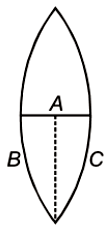1.

2.

3.

4.

Concept Questions :-

Lenses
High Yielding Test Series + Question Bank - NEET 2020

Difficulty Level:

A light beam containing red, yellow and blue color, falls on the interface of glass air as shown. If the yellow light is just totally internally reflected, then emergent ray in air contains-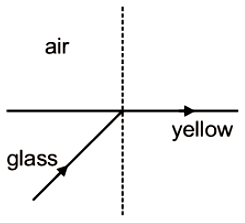1.  Both red and blue color

2.  Only red color

3.  Only blue color

4.  No color

Concept Questions :-

Total internal reflection
High Yielding Test Series + Question Bank - NEET 2020

Difficulty Level:

Focal lengths of objective and eyepiece of a compound microscope are 2 cm and 6.25 cm respectively. An object AB is placed at a distance of 2.5 cm from the objective which forms the image B'A' as shown in the figure. Maximum magnifying power in this case is :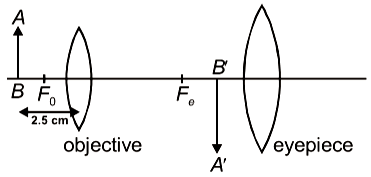1.  10

2.  20

3.  5

4.  25

Concept Questions :-

Simple and compound microscope
High Yielding Test Series + Question Bank - NEET 2020

Difficulty Level:

Two identical equiconvex thin lenses each of focal lengths 20 cm, made of material of refractive index 1.5 are placed coaxially in contact as shown. Now, the space between them is filled with a liquid of refractive index 1.5. The equivalent power of this arrangement will be: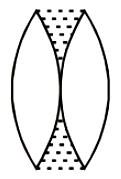1.  + 5D

2.  Zero

3.  + 2.5D

4.  + 0.5D

Concept Questions :-

Lensmakers' formula
High Yielding Test Series + Question Bank - NEET 2020

Difficulty Level:

The length of an astronomical telescope adjusted for parallel light is 90 cm. If the magnifying power of the telescope is 17, then the focal length of eyepiece and objective are respectively

1.  10 cm and 80 cm

2.  85 cm and 5 cm

3.  70 cm and 20 cm

4.  5 cm and 85 cm

Concept Questions :-

Telescope
High Yielding Test Series + Question Bank - NEET 2020

Difficulty Level:

If the wavelength of light used is halved and the numerical aperture of the compound microscope is doubled, then its resolving power will

1. Remain unchanged

2. Doubled

3. Halved

Concept Questions :-

Resolution of Optical devices
High Yielding Test Series + Question Bank - NEET 2020

Difficulty Level:

In the situation shown, the incident monochromatic ray retraces its path after its incidence on the silvered surface. The speed of light inside the prism will be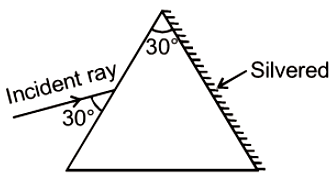1.

2.

3.

4.

Concept Questions :-

Prisms